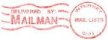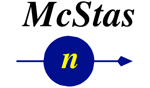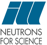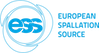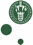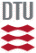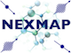McStas: Lens Component

# The `Lens` Component

Refractive lens with absorption, incoherent scattering and surface imperfection.

## Identification

• Author: C. Monzat/E. Farhi/S. Desert/G. Euzen
• Origin: ILL/LLB
• Date: 2009
• Version: \$Revision 1.0\$ - differs from 1.12b version only by corrected typo in author name

## Description

```Refractive Lens with absorption, incoherent scattering and surface imperfection.
Geometry may be:
spherical (use r1 and r2 to specify radius of curvature),
planar (use phiy1 and phiy2 to specify rotation angle of plane w.r.t beam)
or parabolic (use focus1 and focus2 as optical focal length).
The lens cross-section is seen as a 2*radius disk from the beam Z axis.
Usually, you should stack more than one of these to get a significant effect
on the neutron beam, so-called 'compound refractive lens'.
The focal length for N lenses with focal 'f' is f/N.

Common material:
MgF2: density=3.148,weight=62.3018,sigma_coh=11.74,sigma_inc=0.0816,sigma_abs=0.0822
diamond: density=3.52,weight=12.01,sigma_coh=5.551,sigma_inc=0.001,sigma_abs=0.0035
Quartz/silica:density=2.53,weight=60.08,sigma_coh=10.625,sigma_inc=0.0056,sigma_abs=0.1714
perfluoropolymer

Example: Lens(r1=0.025,r2=0.025, thickness=0.001,radius=0.0150)

%BUGS
parabolic shape is not fully validated yet, but should do still...

```

## Input parameters

Parameters in boldface are required; the others are optional.
Name Unit Description Default
r1 m radius of the first circle describing the lens. r>0 means concave face, r<0 means convex, r=0 means plane 0
r2 m radius of the second circle describing the lens r>0 means concave face, r<0 means convex, r=0 means plane 0
focus1 m focal of the first parabola describing the lens 0
focus2 m focal of the second parabola describing the lens 0
phiy1 deg angle of plane1 (r1=0) around y vertical axis 0
phiy2 deg angle of plane2 (r2=0) around y vertical axis 0
thickness m thickness of the lens between its two surfaces 0.001
radius m radius of the lens section, e.g. beam size 0.015
sigma_coh barn coherent cross section 11.74
sigma_inc barn incoherent cross section 0.0816
sigma_abs barn thermal absorption cross section 0.0822
density g/cm3 density of the material for the lens 3.148
weight g/mol molar mass of the material 62.3018
p_interact 1 MC Probability for scattering the ray; otherwise transmit 0.1
focus_aw deg vertical angle to forward focus after scattering 10
focus_ah deg horizontal angle to forward focus after scattering 10
RMS Angs root mean square roughness of the surface 0

## Output parameters

Name Unit Description Default
rho
bc
my_s
my_a_v
mean_n
events

## Links

Generated automatically by McDoc, Peter Willendrup <peter.willendrup@risoe.dk> / Tue Aug 10 11:45:54 2010

Last Modified: Thursday, 21-Mar-2013 13:57:51 CET